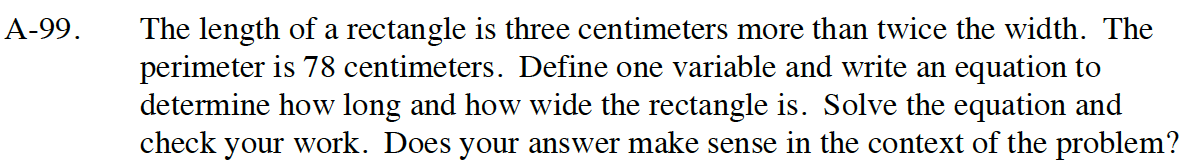Home > CC4 > Chapter A > Lesson A.1.9 > ProblemA-99

A-99.

The length of a rectangle is three centimeters more than twice the width. The perimeter is 78 centimeters. Define one variable and write an equation to determine how long and how wide the rectangle is. Solve the equation and check your work. Does your answer make sense in the context of the problem? Homework Help ✎Try making a table.

Remember that the perimeter is the sum of ALL the sides. Try writing an equation for this situation.

2L + 2W = 78 cm

Remember that the length is three centimeters more than twice the width.That is, L = 3 + 2W.

Length = 27 cm
Width = 12 cm

Make sure you showed your work. Remember that you can always check your answer.